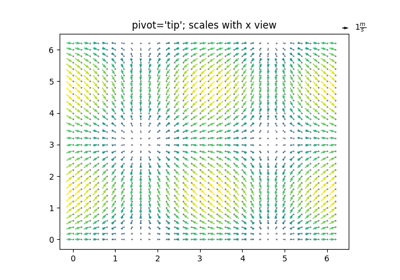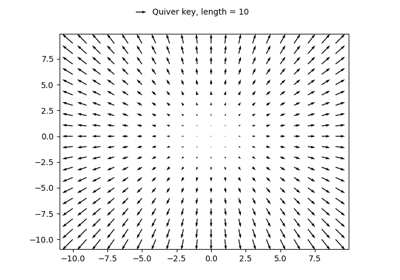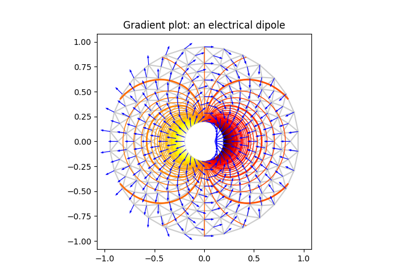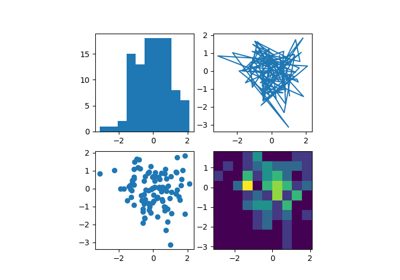# matplotlib.pyplot.quiver¶

matplotlib.pyplot.quiver(*args, data=None, **kw)[source]

Plot a 2D field of arrows.

Call signature:

quiver([X, Y], U, V, [C], **kw)


Where X, Y define the arrow locations, U, V define the arrow directions, and C optionally sets the color.

Arrow size

The default settings auto-scales the length of the arrows to a reasonable size. To change this behavior see the scale and scale_units parameters.

Arrow shape

The defaults give a slightly swept-back arrow; to make the head a triangle, make headaxislength the same as headlength. To make the arrow more pointed, reduce headwidth or increase headlength and headaxislength. To make the head smaller relative to the shaft, scale down all the head parameters. You will probably do best to leave minshaft alone.

Arrow outline

linewidths and edgecolors can be used to customize the arrow outlines.

Parameters:
X, Y1D or 2D array-like, optional

The x and y coordinates of the arrow locations.

If not given, they will be generated as a uniform integer meshgrid based on the dimensions of U and V.

If X and Y are 1D but U, V are 2D, X, Y are expanded to 2D using X, Y = np.meshgrid(X, Y). In this case len(X) and len(Y) must match the column and row dimensions of U and V.

U, V1D or 2D array-like

The x and y direction components of the arrow vectors.

They must have the same number of elements, matching the number of arrow locations. U and V may be masked. Only locations unmasked in U, V, and C will be drawn.

C1D or 2D array-like, optional

Numeric data that defines the arrow colors by colormapping via norm and cmap.

This does not support explicit colors. If you want to set colors directly, use color instead. The size of C must match the number of arrow locations.

units{'width', 'height', 'dots', 'inches', 'x', 'y' 'xy'}, default: 'width'

The arrow dimensions (except for length) are measured in multiples of this unit.

The following values are supported:

• 'width', 'height': The width or height of the axis.
• 'dots', 'inches': Pixels or inches based on the figure dpi.
• 'x', 'y', 'xy': X, Y or $$\sqrt{X^2 + Y^2}$$ in data units.

The arrows scale differently depending on the units. For 'x' or 'y', the arrows get larger as one zooms in; for other units, the arrow size is independent of the zoom state. For 'width or 'height', the arrow size increases with the width and height of the axes, respectively, when the window is resized; for 'dots' or 'inches', resizing does not change the arrows.

angles{'uv', 'xy'} or array-like, optional, default: 'uv'

Method for determining the angle of the arrows.

• 'uv': The arrow axis aspect ratio is 1 so that if U == V the orientation of the arrow on the plot is 45 degrees counter-clockwise from the horizontal axis (positive to the right).

Use this if the arrows symbolize a quantity that is not based on X, Y data coordinates.

• 'xy': Arrows point from (x, y) to (x+u, y+v). Use this for plotting a gradient field, for example.

• Alternatively, arbitrary angles may be specified explicitly as an array of values in degrees, counter-clockwise from the horizontal axis.

In this case U, V is only used to determine the length of the arrows.

Note: inverting a data axis will correspondingly invert the arrows only with angles='xy'.

scalefloat, optional

Number of data units per arrow length unit, e.g., m/s per plot width; a smaller scale parameter makes the arrow longer. Default is None.

If None, a simple autoscaling algorithm is used, based on the average vector length and the number of vectors. The arrow length unit is given by the scale_units parameter.

scale_units{'width', 'height', 'dots', 'inches', 'x', 'y', 'xy'}, optional

If the scale kwarg is None, the arrow length unit. Default is None.

e.g. scale_units is 'inches', scale is 2.0, and (u, v) = (1, 0), then the vector will be 0.5 inches long.

If scale_units is 'width' or 'height', then the vector will be half the width/height of the axes.

If scale_units is 'x' then the vector will be 0.5 x-axis units. To plot vectors in the x-y plane, with u and v having the same units as x and y, use angles='xy', scale_units='xy', scale=1.

widthfloat, optional

Shaft width in arrow units; default depends on choice of units, above, and number of vectors; a typical starting value is about 0.005 times the width of the plot.

Head width as multiple of shaft width.

Head length as multiple of shaft width.

minshaftfloat, optional, default: 1

Length below which arrow scales, in units of head length. Do not set this to less than 1, or small arrows will look terrible!

minlengthfloat, optional, default: 1

Minimum length as a multiple of shaft width; if an arrow length is less than this, plot a dot (hexagon) of this diameter instead.

pivot{'tail', 'mid', 'middle', 'tip'}, optional, default: 'tail'

The part of the arrow that is anchored to the X, Y grid. The arrow rotates about this point.

'mid' is a synonym for 'middle'.

colorcolor or color sequence, optional

Explicit color(s) for the arrows. If C has been set, color has no effect.

This is a synonym for the PolyCollection facecolor parameter.

Other Parameters:
**kwargsPolyCollection properties, optional

All other keyword arguments are passed on to PolyCollection:

Property Description
agg_filter a filter function, which takes a (m, n, 3) float array and a dpi value, and returns a (m, n, 3) array
alpha float or None
animated bool
antialiased or aa or antialiaseds bool or sequence of bools
array ndarray
capstyle {'butt', 'round', 'projecting'}
clim (vmin: float, vmax: float)
clip_box Bbox
clip_on bool
clip_path Patch or (Path, Transform) or None
cmap colormap or registered colormap name
color color or sequence of rgba tuples
contains callable
edgecolor or ec or edgecolors color or sequence of colors or 'face'
facecolor or facecolors or fc color or sequence of colors
figure Figure
gid str
hatch {'/', '\', '|', '-', '+', 'x', 'o', 'O', '.', '*'}
in_layout bool
joinstyle {'miter', 'round', 'bevel'}
label object
linestyle or dashes or linestyles or ls {'-', '--', '-.', ':', '', (offset, on-off-seq), ...}
linewidth or linewidths or lw float or sequence of floats
norm Normalize
offset_position {'screen', 'data'}
offsets array-like (N, 2) or (2,)
path_effects AbstractPathEffect
picker None or bool or float or callable
pickradius unknown
rasterized bool or None
sketch_params (scale: float, length: float, randomness: float)
snap bool or None
transform Transform
url str
urls List[str] or None
visible bool
zorder float

quiverkey
## Examples using matplotlib.pyplot.quiver¶